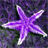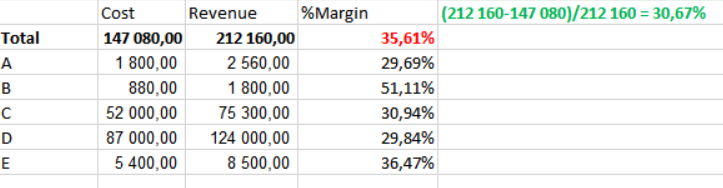# QlikView App Dev

Discussion Board for collaboration related to QlikView App Development.

Announcements
QlikWorld 2023, a live, in-person thrill ride. Save \$300 before February 6: REGISTER NOW!
cancel
Showing results for
Did you mean:Contributor III

## Problem with total of percentage

Hello,

I work with Qlikview 11.

I have a problem with the result of the total expression when it corresponds to a percentage calculation.

Here my example :

Calculation of %Margin = (Revenue - Cost)/RevenueWhen I use the "Average" calculation for the total of the expression, I obtain 35.61% but I would like 30.67% which is obtained with the total values of Cost and Revenue (see green information).

Can someone help me to solve this problem ?

17 RepliesMVP

Is this in the straight table? May be try to use Expression Total instead of Avg Total ModeMaster

do you use any aggregation like sum in Your Expression?

sum((Revenue) - sum(Cost))/sum(Revenue)?Contributor III
Author

Yes I use a straight table. I can't use Total because QV makes the sum of percentage.MVP

Really? Can you share a sample to show this issue?Contributor III
Author

In fact, the expression is like this :

if (qty <>0,

(
(
sum({<Date_fact = {">\$(DATE_REF)<=\$(DATE_REF)"}>} Revenue
) - (
Unit_cost * sum({< Date_fact = {">\$(DATE_REF)<=\$(DATE_REF)"} >} Qty ) ) )
/
(
sum({<Data_fact = {">\$(DATE_REF)<=\$(DATE_REF)"}>} Revenue) )
))

DATE_REF is selected in a calendar by the user.

Date_fact is the invoice date

Unit_cost is a variable defined like this :

MAX({< begining_date = {">=\$(DATE_REF)"}, end_date = {"<=\$(DATE_REF_YYYYMMDD)"}, price_type={"80"}>} PRICE)Partner - Champion III

There may be side-effects at play in your document. Better post an example document that exhibits this weird behavior.Master

Hi Christelle,

Your problem is that you start the Expression With an if. This will work on every row in Your chart, but in total you will have all values regardless of Your if statment if (qty <>0. One way to solve this is to use aggr() function.Use the Dimension from where you get A,B,C... Give this Expression a try, remember to change to Your used Dimension.

sum(aggr(if (qty <>0,

(
(
sum({<Date_fact = {">\$(DATE_REF)<=\$(DATE_REF)"}>} Revenue
) - (
Unit_cost * sum({< Date_fact = {">\$(DATE_REF)<=\$(DATE_REF)"} >} Qty ) ) )
/
(
sum({<Data_fact = {">\$(DATE_REF)<=\$(DATE_REF)"}>} Revenue) )
)) ,Dimension))Contributor III
Author

Hi Staffan,

I try your expression but it does'nt work.

When I use the "total of expression" option or  the "sum of lines", I obtain the sum of percentages.

I write :

=sum(aggr((If(\$(SommeQté)<>0 OR GetSelectedCount(%_Etablissement_Filliale) = 0,
(
(
sum({<UCIVDT = {">\$(DATE_REF_1_YYYYMMDD)<=\$(DATE_REF_YYYYMMDD)"}>} UCUCOS
) - (
\$(CSSN)sum({< UCIVDT = {">\$(DATE_REF_1_YYYYMMDD)<=\$(DATE_REF_YYYYMMDD)"} >} UCIVQT ) ) )
/
(
sum({<UCIVDT = {">\$(DATE_REF_1_YYYYMMDD)<=\$(DATE_REF_YYYYMMDD)"}>} UCUCOS) )
)),
[Code article] ))Master

ok,

Expression total is to prefer here I'll think.Community Browser